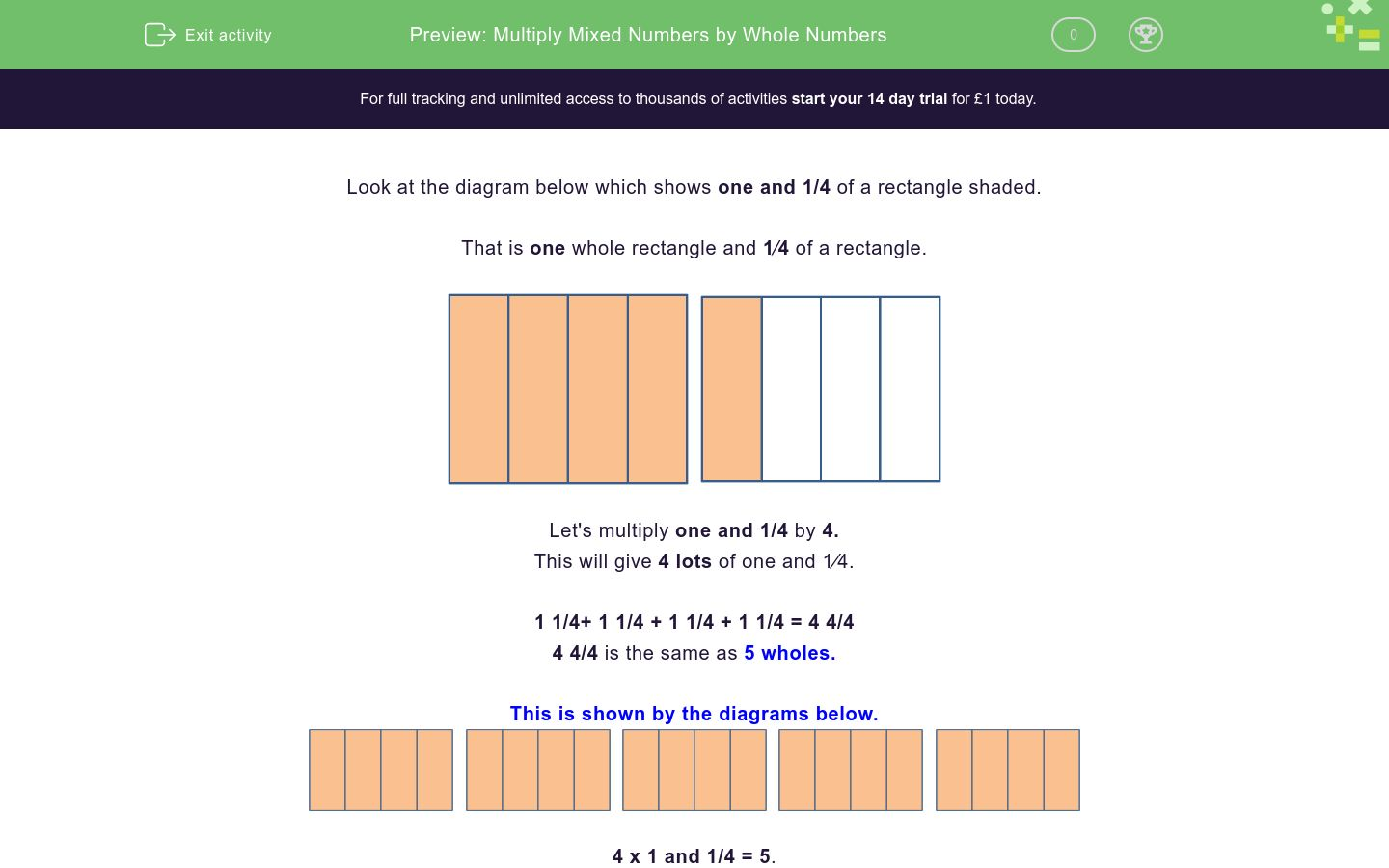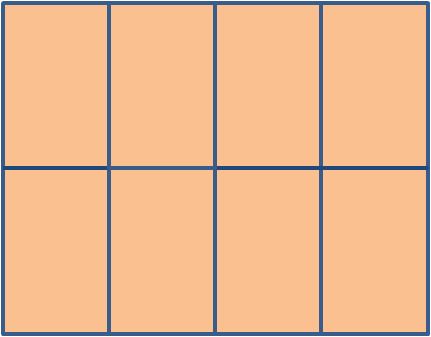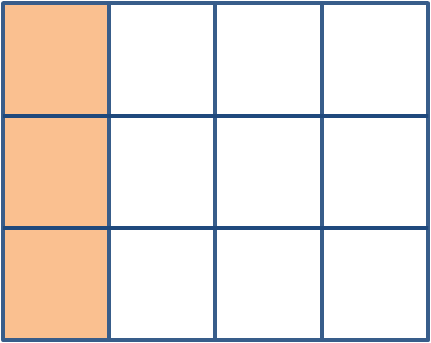# Multiply Mixed Numbers by Whole Numbers

In this worksheet, students will be asked to multiply mixed numbers by whole numbers.Key stage:  KS 2

Curriculum topic:   Number: Fractions, Decimals and Percentages

Curriculum subtopic:   Multiply Fractions

Difficulty level:### QUESTION 1 of 10

Look at the diagram below which shows one and 1/4 of a rectangle shaded.

That is one whole rectangle and 1⁄4 of a rectangle.Let's multiply one and 1/4 by 4.

This will give 4 lots of one and 1⁄4.

1 1/4+ 1 1/4 + 1 1/4 + 1 1/4 = 4 4/4

4 4/4 is the same as 5 wholes.

This is shown by the diagrams below.4 x 1 and 1/4 = 5.Use the diagrams to help solve the multiplication sum...

5 x 1 1/4  = ?

6 1/4

5 1/4

6

6 1/8Use the diagram to help solve the multiplication sum below.

3 x 1 2/6 =

4

5

3 2/6

3 4/6Use the diagram to help solve the multiplication sum below.

2 x 1 2/8 = ?

2 4/8

2 2/8

2 1/8

3Use the diagram in order to work out the equation below.

3 x 2 2/8 =?

2 4/8

2 2/8

2 1/8

3Use the diagram to work out and complete the equation below.

2 x 1 3/12 = ?

2 4/8

2 2/8

2 1/8

3Use the diagram to work out and complete the equation below.

3 x 3 1/4 = ?

2 4/8

2 2/8

2 1/8

3Use the diagram to work out and complete the equation below.

4 x 1 1/6 = ?Use the diagram to work out and complete the equation below.

2 x 2 3/8 =?

At a birthday party, some children share a pizza.

Each child eats 3/16 of the pizza.

How much pizza do they eat in total?A cake's ingredient list requires 1 1/8 of a bag of flour in order to make one large cake.

A baker needs to make 7 cakes.

How much flour will she need in total?• Question 1Use the diagrams to help solve the multiplication sum...

5 x 1 1/4  = ?

6 1/4
EDDIE SAYS
How did you find this first question? 5 x 1 1/4 = 6 1/4 It helps to multiply all of the whole numbers first. 5 x 1 = 5 Then multiply the fractions 5 x 1/4 = 1 1/4 5 + 1 1/4 = 6 1/4
• Question 2Use the diagram to help solve the multiplication sum below.

3 x 1 2/6 =

4
EDDIE SAYS
Hopefully, this second question is helping you to get the hang of it. 3 x 1 2/6 = 4 Remember, it helps to multiply all of the whole numbers first. 3 x 1 = 3 Then multiply the fractions 3 x 2/6 = 6/6 6/6 is the same as one whole. Add together 3 + 1 = 4
• Question 3Use the diagram to help solve the multiplication sum below.

2 x 1 2/8 = ?

2 4/8
EDDIE SAYS
Did you choose the correct answer? 2 x 1 2/8 = 2 4/8 Remember, it can help us to multiply all of the whole numbers first. 2 x 1 = 2 Then multiply the fractions 2 x 2/8 = 4/8 Add together 2 + 4/8 = 2 4/8
• Question 4Use the diagram in order to work out the equation below.

3 x 2 2/8 =?

EDDIE SAYS
When we multiply mixed numbers by whole numbers, it can help to multiply the whole numbers first... 3 x 2 = 6 Then multiply the fractions 2/8 x 3 = 6/8 And finally, add both together = 6 6/8
• Question 5Use the diagram to work out and complete the equation below.

2 x 1 3/12 = ?

EDDIE SAYS
As before, don't forget that when we multiply mixed numbers by whole numbers, it can help to multiply the whole numbers first, 2 x 1 = 2 Multiply the fractions 2 x 3/12 = 6/12 Finally, add the two together = 2 6/12
• Question 6Use the diagram to work out and complete the equation below.

3 x 3 1/4 = ?

EDDIE SAYS
Again, when we multiply mixed numbers by whole numbers, it can help to multiply the whole numbers first. 3 x 3 = 9 Then multiply the fractions 3 x 1/4 = 3/4 And finally, add them together = 9 3/4
• Question 7Use the diagram to work out and complete the equation below.

4 x 1 1/6 = ?

4 4/6
EDDIE SAYS
This time you had to work out every part of the answer independently! 4 x 1 1/6 = 4 4/6 4 x 1 = 4 4 x 1/6 = 4/6
• Question 8Use the diagram to work out and complete the equation below.

2 x 2 3/8 =?

4 6/8
EDDIE SAYS
Again, it was over to you to work out every part of the answer on your own. 2 x 2 3/8 = ? 2 x 2 = 4 2 x 3/8 = 6/8 You're getting good at this!
• Question 9

At a birthday party, some children share a pizza.

Each child eats 3/16 of the pizza.

How much pizza do they eat in total?15/16
EDDIE SAYS
The quickest way to find out how much pizza was eaten is to carry out a multiplication sum. 5 x 3/16 = 15/16 The denominator of the fraction stays the same. The numerator is multiplied by 5.
• Question 10

A cake's ingredient list requires 1 1/8 of a bag of flour in order to make one large cake.

A baker needs to make 7 cakes.

How much flour will she need in total?7 7/8
EDDIE SAYS
The quickest way to find out how much flour is needed is to carry out a multiplication sum. 7 x 1 1/8 = 7 7/8 7 x 1 = 7 7 x 1/8 = 7/8 Great work, you've completed another activity! How about attempting another one so you feel super confident?
---- OR ----

Sign up for a £1 trial so you can track and measure your child's progress on this activity.

### What is EdPlace?

We're your National Curriculum aligned online education content provider helping each child succeed in English, maths and science from year 1 to GCSE. With an EdPlace account you’ll be able to track and measure progress, helping each child achieve their best. We build confidence and attainment by personalising each child’s learning at a level that suits them.

Get started